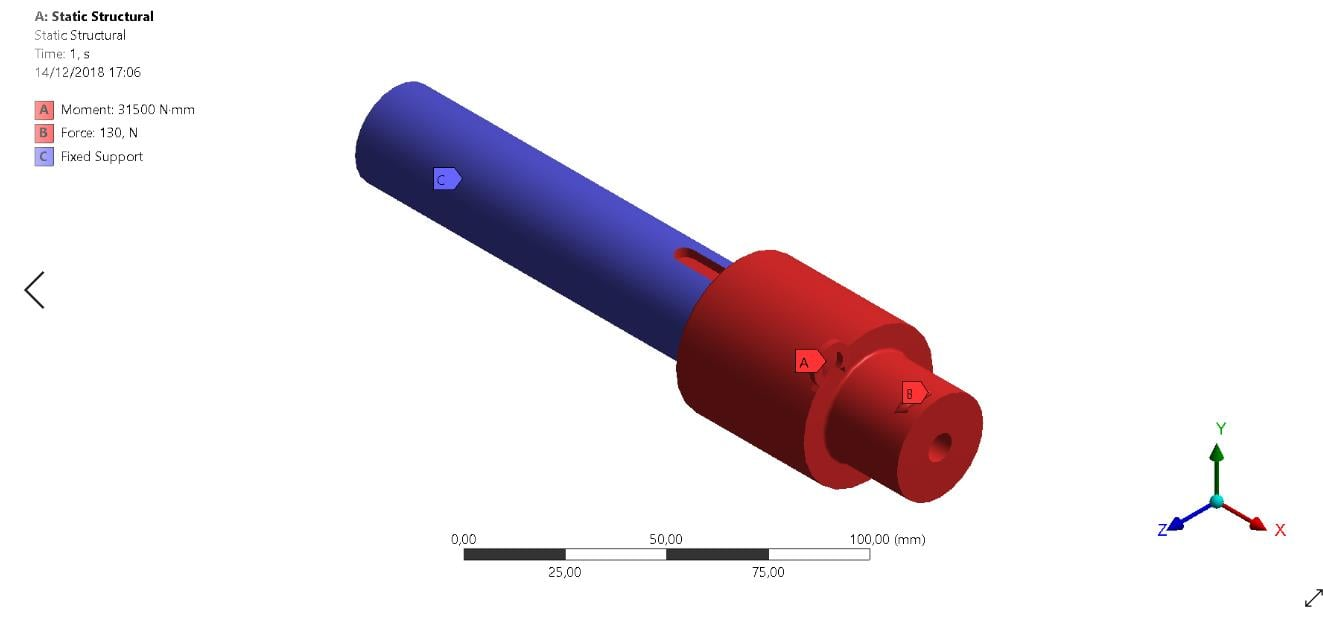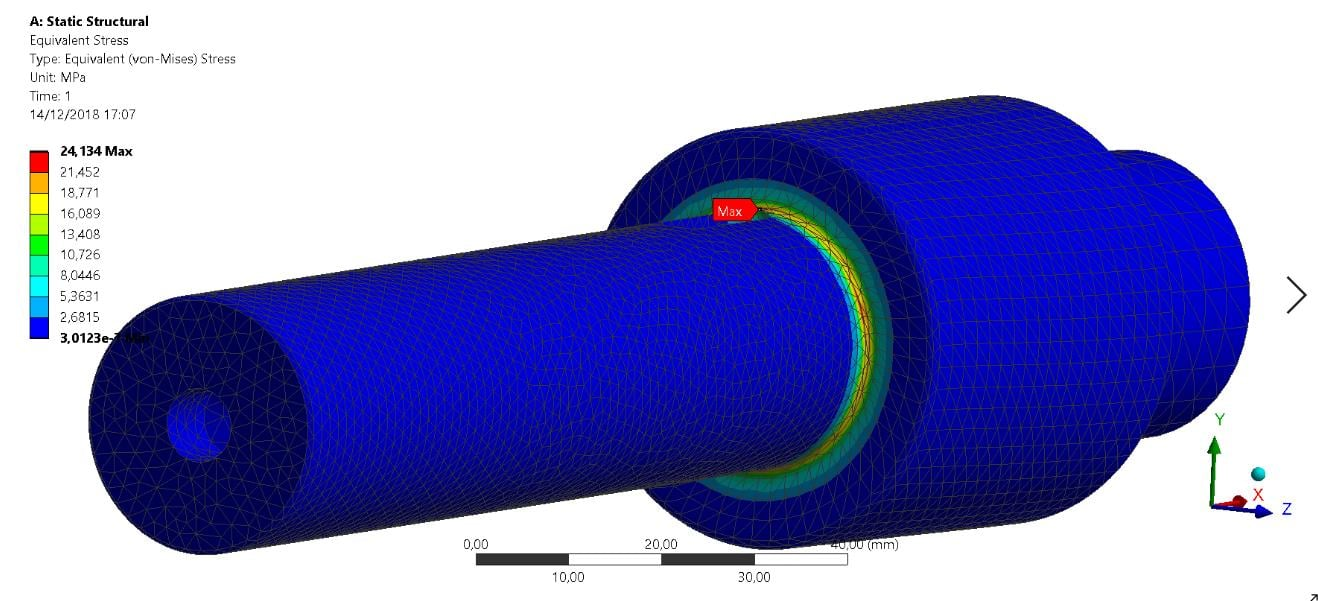## General Mechanical

•salocin155
Subscriber

Hi im trying to simulate a shaft with a torque and a axial force on one site, i already calculated the minium diameter for the shaft acording do the book Mechanical Engineering Design Shingley, and i got a value of minimum of 10mm shaft so i choose a 30mm shaft, having a  factory safety of 3. I want to simulate that shaft just to prove that my shaft wont break. So i simulated that shaft with a 35Nm torque, and an axial force of 130N, put the problem is im only getting a 24MPa Von Mises with those forces and that means my safety factor acording to the simulation would be something like 20. Dont know if my boundary conditions are alright or if the result is fine and i just cant understand that big of an diference between the choosen safety factor and the simlated one.

Boundarie Condition: I aplied fixed suport on the part that the shaft in insite the worm gearbox, torque on all the shaft and axial force on the part where is gona be an tagencial force acting .•peteroznewman
Subscriber

You say the minimum shaft diameter from equations in Shigley gives you 10 mm. Are those equations considering fatigue failure?

If you know the material Yield Strength or Fatigue Strength and have an equation that takes a diameter and calculates the maximum stress, then you adjust the diameter until the Maximum Stress = Strength. Now you have determined a design with a Safety Factor of 1.0

You need to put in slightly larger diameters into that equation until Maximum Stress = Strength/3 to get a Safety Factor of 3.0  You don't multiply the diameter by 3 to get a Safety Factor of 3.0, you will likely get a Safety Factor of 20 like you did.

## Boundary Conditions for a Shaft with Two Gears

The shaft has two bearings. Divide the face of the shaft at four planes, at each side of each bearing. Maybe one of the bearings is that end cylinder so you don't need to four planes, just two. Use those two bearing faces and put a remote displacement on each face separately. One of those bearings resists the axial force of the worm gear. On that remote displacement, set X,Y,Z at 0 and leave all rotations Free. On the other remote displacement scoped to the other bearing face, set Y,Z at 0 and leave all others Free.

The shaft has a wormgear somewhere on the shaft and larger gear represented by the larger diameter, so you already have a face for the larger gear. Use two more planes on the shaft to divide the shaft face to the length of the wormgear and put a remote displacement on that new face, set Rotation X at 0 and leave all others Free. Put a remote force on the face that represents the larger gear. I assume the gear has a helix, and that is where the axial force is coming from. You can edit the coordinates of the location of the remote force and move it from the shaft axis out to the pitch circle radius of the larger gear. Now you can put the two coordinates of force into that detail window. An axial (X) force and a tangential (Z) force to represent the moment. You calculate the tangential force from moment/radius where radius is the distance of the remote point from the axis. If it is a helical gear, there is a third force component and that is radial due to the pressure angle of the tooth to the mating gear. All three components acting at the pitch circle create a bending moment on the shaft that creates stress at the shoulder you show.

Regards,
Peter

•salocin155
Subscriber

Ty vm, might have used the safety factor wrong will do again my maths then go back to the simulation.